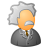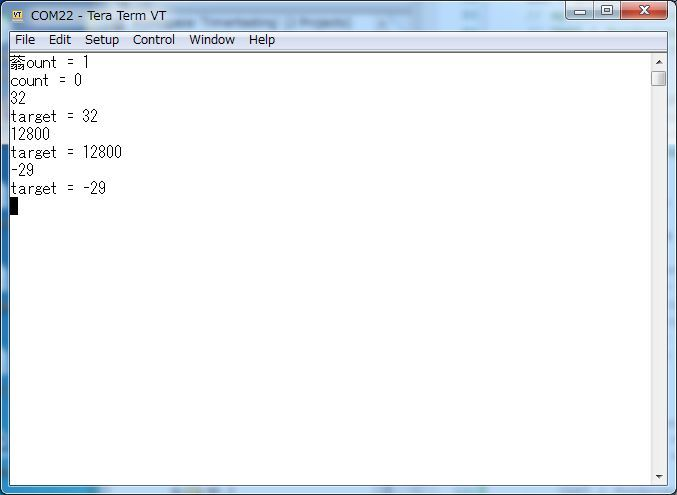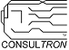# PSoC™ 5, 3 & 1 Forum DiscussionsLevel 1
Level 1

# How can I get an integer from serial port?

I am trying to send a integer in bytes from python to the PSoC to update the target position of our PID control. The PID control is meant to move the motor to its desired position. But every time I send a byte array from python to PSoC using uart and rx_interrupt, it will go back to zero. I don't know why is it the case and how can I fix this problem? I have attached my workspace and my python file below. I appreciate it so much if you can help me with it! Thank you so much!

Sincerely,

Caesar

11 RepliesModeratorModerator# Re: How can I get an integer from serial port?

Hi Caesar,

What is the meaning of "go back to zero" ?

Could you try using a PC UART tool to check if PSoC UART can work well?

Thanks,

RyanLevel 1
Level 1

# Re: How can I get an integer from serial port?

Hi Ryan,

It means every time I tried to send an integer in bytes through the uart, my motor will go to its original position, which is zero.

The Uart is working since I can send a letter to the PSoC and it will respond correctly. I don't know how to transfer an integer to it though.

Sincerely,

CaesarLevel 9
Level 9# Re: How can I get an integer from serial port?

Dear Caesar-san,

You checked interrupts for UART_1

and

TX - On FIFO Empty

So every time you send something via uart,  isr_2 is activated,

then your "MY_ISR_2" is called then it assigns target when both data and data is 0,

so if all data, data, data, data are 0, target will be 0,

and I think this is what you are seeing.

How about un-checking TX - On FIFO Empty interrupt in UART_1?

Best Regards,

3-May-2019

Motoo TanakaLevel 1
Level 1

# Re: How can I get an integer from serial port?

Hi Motoo,

I am wondering what do you mean by

"then your "MY_ISR_2" is called then it assigns target when both data and data is 0,

so if all data, data, data, data are 0, target will be 0"

How can I get all of them and then convert them back to integer?

Sincerely,

CaesarLevel 9
Level 9# Re: How can I get an integer from serial port?

Dear Caesar-san,

I'm sorry for my poor description.

What I wanted to inform you was that as you enabled interrupt for both

RX - On Byte Received and TX - On FIFO Empty in the UART_1 Configuration,

even when you are writing to UART_1, MY_ISR_2 is called when TX (sending) buffer gets empty

which is normal condition after sending message back.

So only disabling TX - On FIFO Empty interrupt in UART_1 may take care of the problem.

The rest of my description was to make sure that a condition which makes "target"  to 0

can take place if all of data ~ data are 0, when you send message back to UART_1.

As I briefly read your code, I think converting short to two uint8_t seems to be fine,

but to avoid the case like this you may want to assign data and data non zero values

as packet signature to check if these 4 bytes are really the packet.

Best Regards,

3-May-2019

Motoo TanakaLevel 1
Level 1

# Re: How can I get an integer from serial port?

Hi Motoo,

I am not sure if my code of receiving data (from line 147 to 164) is correct. Is there a way to transmit an integer from laptop side using UART and receiving on PSoC side and then convert it back to an integer?

Sincerely,

CaesarLevel 9
Level 9# Re: How can I get an integer from serial port?

Dear Caesar-san,

As I'm not fluent with python, I tried some experimental change to your program so that I can use TeraTerm to communicate with your program.If I enter some integer number, which can be positive or negative,

program returns the target if it was changed from the previous value.

Meantime if "cnt" is changed it will report the value in the main loop instead of the ISR_1.

I hope that at least you can see how your system react to the given value.

And in case the response is not adequate, we can go back to the packet style UART communication.

main.c

============================

#include "project.h"

#include "stdio.h"

#include "stdlib.h"

volatile short int cnt = 0;

volatile signed short int target = 1000;

volatile short int prev_count = 0 ;

volatile signed short int prev_target = 0 ;

volatile uint8_t counts = { 0, 0, 0, 0, 0, 0 } ;

int error = 0;

int last_error = 0;

int derivative = 0;

int integral = 0;

int duty_cycle = 0;

char transmitbuffer; // transmit buffer taken out for testing purposes

char ch;

int convert_target;

#define CR '\r'

#define LF '\n'

#define TAB '\t'

#define SPACE ' '

inline int is_delimitar(uint8_t c)

{

return((c == CR)||(c == LF)||(c == TAB)||(c == SPACE)) ;

}

#define RX_BUF_LEN 32

char str ; /* print buffer */

void print(char *str)

{

UART_1_PutString(str) ;

}

volatile uint8_t rx_buf[RX_BUF_LEN] ;

volatile int     rx_index = 0 ;

// The following function is a non-general implementation of

// finding out how long the sending array is.

// Needed near the duty cycle calculaion

int bytesCharArrayNeeds(int dutyCycle) {

int arrSize = 1;

if (dutyCycle >= 10) {

arrSize += 1;

}

if (dutyCycle >= 100) {

arrSize += 1;

}

if (dutyCycle >= 1000) {

arrSize += 1;

}

if (dutyCycle >= 10000) {

arrSize += 1;

}

return arrSize;

}

// only works for a two byte integer, no nothing larger than 16000-ish

uint8 firstByte(short int number) {

return (number & 0xFF00) >> 8;

}

uint8 forthByte(short int number) {

return 0x00FF & number;

}

signed short int combineBytes(uint8 firstByte, uint8 secondByte) {

return ((firstByte << 😎 & 0xFF00) | (0x00FF & secondByte);

}

CY_ISR(MY_ISR_1) {

uint8_t int_flag ;

//target = (int) UART_1_GetChar();

// Get current position

// sprintf(transmitbuffer, "count: %d \n\r", cnt);

// UART_1_PutString(transmitbuffer);

//UART_1_PutArray((uint8 *) cnt, sizeof(cnt)); // Since it needs a string array,

// we cast to pointer

// byteCount shount not be user defined

// uint8 charArraySize = bytesCharArrayNeeds(cnt);

// char transmission[charArraySize];

// sprintf(transmission, "%d", cnt); // sPrintF family uses u as unsigned, not d

// UART_1_PutString(transmission);

if (multitasking % 2 == 0) { // this takes place every 20ms, I wonder if we should print here

#if 0

if (cnt != prev_count) {

int_flag = CyEnterCriticalSection() ;

sprintf(str, "count = %d\n", cnt) ;

CyExitCriticalSection(int_flag) ;

print(str) ;

prev_count = cnt ;

}

#endif

#if 0

if (cnt != prev_count) {

counts = firstByte(cnt) ;

counts = forthByte(cnt) ;

}

UART_1_PutArray(counts, 6) ;

#endif

#if 0

uint8 counts;

counts = 0x00;

counts = 0x00;

counts = firstByte(cnt);

counts = forthByte(cnt);

counts = 0x00;

counts = 0x00;

uint8* p = counts;

UART_1_PutArray(p, sizeof(counts));

#endif

}

// Calculate the error

error = target - cnt;

// sprintf(transmitbuffer, "error: %d \r\n", error);

// UART_1_PutString(transmitbuffer);

//Calculate the integral

integral = integral + error;

// Calculate the Derivative

derivative = error - last_error;

//Calculate the control variable

duty_cycle = 100 * error + 1 * integral + 50 * derivative;

// Limit the control variable within 100

if (duty_cycle > 32000){duty_cycle = 32000;}

else if (duty_cycle < -32000){duty_cycle = -32000;}

if (duty_cycle < 50 && duty_cycle > -50){

PWM_1_WriteCompare(0);

}

// If the control variable is positive, run the motor clockwise

if (duty_cycle > 0){

Direction_1_1_Write(0);

Direction_1_2_Write(1);

PWM_1_WriteCompare(duty_cycle);

}

// If the control variable is negative, run the motor counterclockwise

else if (duty_cycle < 0){

Direction_1_1_Write(1);

Direction_1_2_Write(0);

PWM_1_WriteCompare(-1*duty_cycle);

}

// If the control variable is zero, stop the motor

else{

Direction_1_1_Write(0);

Direction_1_2_Write(0);

}

last_error = error;

// Ping-pong buffer/FIFO buffer

// Update button

}

CY_ISR(MY_ISR_2){

char data;

data = UART_1_GetByte();

data = UART_1_GetByte();

data = UART_1_GetByte();

data = UART_1_GetByte();

//data = UART_1_GetByte();

//data = UART_1_GetByte();

if (data == 0x0 && data == 0x0 ) {

LED_Write(1);

LED_Write(0);

uint8 firstByte = data;

uint8 secondByte = data;

target = combineBytes(firstByte, secondByte);

}

// convert_target = (int) ch;

// target += ch;

}

CY_ISR(MY_ISR_2_moto)

{

uint8_t c ;

uint8_t int_flag ;

if (UART_1_GetRxBufferSize()) {

c = UART_1_GetByte() ;

if (is_delimitar(c)) {

rx_buf[rx_index] = (char)0 ;

if (rx_index != 0) {

int_flag = CyEnterCriticalSection() ;

sscanf((const char*)rx_buf, "%hd", &target) ;

CyExitCriticalSection(int_flag) ;

rx_index = 0 ;

rx_buf[rx_index] = 0 ;

}

} else {

rx_buf[rx_index] = c ;

rx_index++ ;

if (rx_index >= RX_BUF_LEN) {

rx_index = 0 ;

}

}

}

}

int main(void)

{

int16_t prev_target ;

/* Place your initialization/startup code here (e.g. MyInst_Start()) */

PWM_1_Start();

UART_1_Start();

Timer_1_Start(); // Configure and enable timer

//  isr_1_Start(); // Start the timer interrupt // StatrEX only will be fine

isr_1_StartEx(MY_ISR_1); // Point to MY_ISR_1 to carry out the interrupt sub-routine of PID control

//   isr_2_Start(); // Start the control variable changing interrupt // StartEx only will be fine

isr_2_StartEx(MY_ISR_2_moto); // Point to moto's MY_ISR_2_moto

//  isr_2_StartEx(MY_ISR_2); // Point to MY_ISR_2 to carry out the interrupt sub-routine of changing target postiion

CyGlobalIntEnable; /* Enable global interrupts. */

prev_target = target ;

for(;;)

{

if (target != prev_target) { // report target if it was changed

sprintf(str, "target = %d\n", target) ;

print(str) ;

prev_target = target ;

}

#if 1

if (cnt != prev_count) { // report cnt if it was changed

sprintf(str, "count = %d\n", cnt) ;

print(str) ;

prev_count = cnt ;

}

#endif

CyDelay(100) ;

}

//uint8 number1 = byteArray

//uint8 number2 = byteArray

}

============================

motoLevel 9
Level 9# Re: How can I get an integer from serial port?

Caesar,

I have not looked at your project at this point.

Please consider the following design issues:

• When you send an integer, is your integer one byte, two bytes or four bytes.  The 'integer' term means not floating but different compilers use integer to mean 1, 2 or 4 bytes.  Hence the confusion.  That's why most compilers recommend using uint8, uint16 and uint32 to be non-ambiguous.  The size of the integer is the last set of numbers.
• If your integer is 2 or more bytes, you have to make sure the endian order is correct.  The ARM (PSoC5) favors little-endian, other systems may favor Big-endian.  Make sure when you send or receive the bytes to form the integer, the order is what you expected.
• Are your integers actual numbers converted to characters before sending?   If so, you may want to check your character-to-number conversion formula in the receiver.
• If you are using printf-like or sprintf-like functions in python, they may be placing a NULL character ('\0') at the end of the string.  If this is occurring maybe your receiving program is interpreting this as a "return to zero" condition.

I'll take a look at your project soon however I'm not familiar with python.

Len

Len
"Engineering is an Art. The Art of Compromise."Level 9
Level 9# Re: How can I get an integer from serial port?

Caesar,

I'm not sure I have the answer you're looking for but this is what I observed in your PSoC project.

• You are dumping an array of 6 bytes.  However bytes 0, 1, 4 and 5 are '0'.  Only bytes 2 and 3 have values.  Is this your intent?

if (multitasking % 2 == 0) {    uint8 counts;    counts = 0x00;    counts = 0x00;    counts = firstByte(cnt);    counts = forthByte(cnt);    counts = 0x00;    counts = 0x00;    uint8* p = counts;    UART_1_PutArray(p, sizeof(counts));

}

• 'cnt' listed above is a 'short' (2-byte) value.  You appear to using little-endian format across the serial link to the receiver of the PID control.  (ie. LSB first, MSB last).
• UART_1_PutArray() is a blocking function if the array size (6 in your design) exceeds the FIFO size (4).  You are entering a blocking function within a ISR.  This is generally not a good idea in pure coding terms.  This means you are blocking ALL other interrupts until you complete the UART_1_PutArray() .
• You have declared a Tx interrupt output "Tx - On FIFO Empty" instead of "Tx - On FIFO Not Full".  This will cause a potential slight delay between the 4th and 5th bytes of the array.  This may or may not be a problem for you.  Using "Tx - On FIFO Not Full" make better use of the FIFO HW of the UART component.   Upon closer look: This may not be an issue since I don't see where you are servicing a UART_1 Tx interrupt in your code.

I can't comment on your Python code due to my unfamiliarity.

Lnn

Len
"Engineering is an Art. The Art of Compromise."Level 1
Level 1

# Re: How can I get an integer from serial port?

Hi Lnn,

I am having a problem of receiving data on PSoC side. However the code for multitasking is for PSoC to send data. The receiving code is

CY_ISR(MY_ISR_2){

char data;

data = UART_1_GetByte();

data = UART_1_GetByte();

data = UART_1_GetByte();

data = UART_1_GetByte();

//data = UART_1_GetByte();

//data = UART_1_GetByte();

if (data == 0x0 && data == 0x0 ) {

LED_Write(1);

LED_Write(0);

uint8 firstByte = data;

uint8 secondByte = data;

target = combineBytes(firstByte, secondByte);

}

// convert_target = (int) ch;

// target += ch;

}

, which is located at 147 to 164 lines. Is it the right way to receive an integer on PSoC? If the integer has multiple bytes while transmitting, how can I implement the receiving data on PSoC side and convert it back to integer?

Sincerely,

CaesarLevel 9
Level 9# Re: How can I get an integer from serial port?

Ceasar,

It appears that your combineBytes() function is converting your two bytes into the proper little-endian format for the short function.

There are two ways to confirm this.

1. If you can tolerate a halt in the CPU, you can run the code in debug mode.
2. Set up a UART to talk to the USB and create some debug data dumps of the 'target' variable.

Len

Len
"Engineering is an Art. The Art of Compromise."